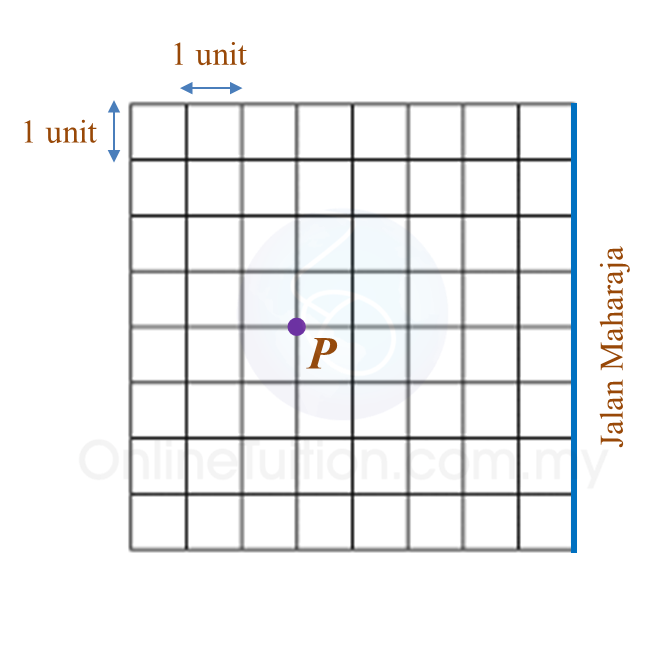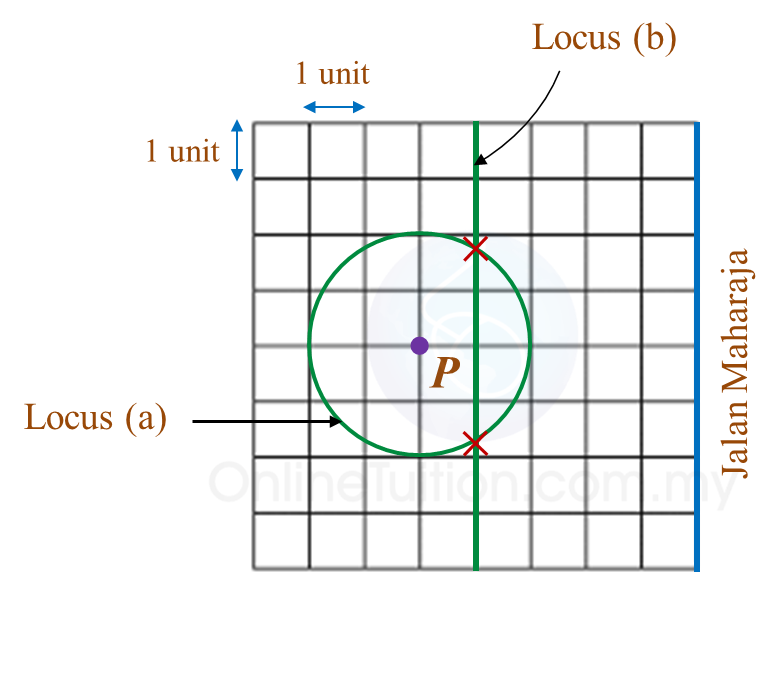# PT3 Mathematics 2017, Question 5

Question 5 (a):
Rajah 5.1 shows two aquariums with an equal number of fish.Based on Diagram 5.1,
(i) write a linear equation.
(ii) find the value of w.

Solution:
(i)
w – 5 = 13

(ii)
w – 5 = 13
w = 13 + 5
w = 18

Question 5 (b):
Faisal has drawn a plan for a treasure hunt game on a grid of equal squares with the scale of 1 unit to 1 metre.
(i) On Diagram 5.2 in the answer space, draw,
(a) the locus of the treasure box if it is always 2 m from the flag pole P.
(b) the locus of the treasure box if it is always 4 m from Jalan Maharaja.
(ii) Hence, mark  $\otimes$  on the spots where the treasure box may be placed to satisfy the both conditions .Solution:Question 5 (c):
In a library, the number of reference books is 1600. The total number of reference books and fictional books is 5200. The ratio of fictional books to reference books is x : 4.
(i) Calculate the value of x.
(ii) The fictional books can be classified into Malay and English fictional books. The number of Malay fictional books is two times the number of English fictional books.
Find the ratio of Malay fictional books to English fictional books to reference books in the lowest term.

Solution:
(i)
$\begin{array}{l}\frac{x}{4}=\frac{5200-1600}{1600}\\ x=\frac{3600}{1600}×4\\ x=9\end{array}$

(ii)